Students must start practicing the questions from CBSE Sample Papers for Class 10 Maths with Solutions Set 10 are designed as per the revised syllabus.

## CBSE Sample Papers for Class 10 Maths Set 10 with Solutions

Time Allowed: 3 Hours
Maximum Marks: 80

General Instructions:

• This Question Paper has 5 Sections A, B, C, D, and E.
• Section A has 20 Multiple Choice Questions (MCQs) carrying 1 mark each.
• Section B has 5 Short Answer-I (SA-I) type questions carrying 2 marks each.
• Section C has 6 Short Answer-ll (SA-II) type questions carrying 3 marks each.
• Section D has 4 Long Answer (LA) type questions carrying 5 marks each.
• Section E has 3 Case Based integrated units of assessment (4 marks each) with sub-parts of the values of 1, 1 and 2 marks each respectively.
• All Questions are compulsory. However, an internal choice in 2 Qs of 2 marks, 2 Qs of 3 marks and 2 Questions of 5 marks has been provided. An internal choice has been provided in the 2 marks questions of Section
• Draw neat figures wherever required. Take π = 22/7 wherever required if not stated.

SECTION – A (20 marks)
(Section – A consists of 20 questions of 1 mark each)

Question 1.
The value of x so that the distance between the points (- 3,4) and (x, – 4) is 10 units is: 
(a) -6, 4
(b) -9, 3
(c) 2, 5
(d) 5, 7
(b) -9, 3

Explanation:
Using distance formula, we have
(x + 3)2 + ( – 4 – 4)2 = 100
⇒ (x + 3)2 = 100 – 64 = 36
⇒ x + 3 = ± 6
⇒ x = – 9, 3.

Question 2.
The mode of a frequency distribution and give the formula used in computing the mode of a grouped frequency distribution.(d) Mode = l + $$\frac{f_1-f_0}{2 f_1-f_0-f_2}$$ × h

Explanation:
The mode of a frequency distribution is the value of the highest frequency.
Formula:
Mode = l + $$\frac{f_1-f_0}{2 f_1-f_0-f_2}$$ × h
Where, l = lower limit of the modal class
h = size of the class-interval
f1 = frequency of the modal class
f0 = frequency of the class preceding the modal class
f2 = frequency of the class succeeding the modal class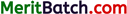Question 3.
The vertices of an equilateral triangle ABC are (0, 0), (0, y) and (3, √3), then the value of y is: 
(a) $$\sqrt{12}$$
(b) $$\sqrt{13}$$
(c) $$\sqrt{14}$$
(d) $$\sqrt{15}$$
(a) $$\sqrt{12}$$

Explanation:
Let the three given points be
O(0, 0), A (0, y) and B (3, 43).
Then OA = AB = OB [∵ AABC is equilateral]
So, using distance formula,
OA = OB
⇒ y = $$\sqrt{3^2+(\sqrt{3})^2}$$
y = $$\sqrt{12}$$

Question 4.
if a hexagon ABCDEF circumscribes a circle, then AB + CD + EF is equal to: 
(a) AB + CD + EF
(b) AF + BC + DE
(c) AF + BD + CE
(d) AC + FB + DE
(b) AF + BC + DE

Explanation:
We know, lengths of tangents drawn from an external point to a circle are equal.
Now, AB + CD + EF = (AP + BP) + (CR + DR) + (ET + FT)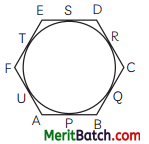= (AU + BQ) + (CQ + DS) + (ES + FU)
= (AU + FU) + (BQ + CQ) + (DS + ES)
= AF + BC + DE or BC + DE + FA.

Question 5.
Three identical cubes each of volume 27 cu cm are joined together end to end. What are the dimensions of the resulting cuboid ? 
(a) 9 cm × 4 cm × 3 cm
(b) 3 cm × 3 cm × 3 cm
(c) 9 cm × 3 cm × 3 cm
(d) 3 cm × 9 cm × 6 cm
(c) 9 cm × 3 cm × 3 cm

Explanation:
Since, volume of each cube is 27 cu. cm, so each cube is of edge 3 cm.
So, dimensions of the resulting cuboid are 9 cm × 3 cm × 3 cm.

Question 6.
If a chord of a circle of radius ‘r’ subtends a right angle at the centre of the circle, then the area of the corresponding segment is: 
(a) $$\frac{\pi r^2}{4}-\frac{1}{2}$$r2
(b) $$\frac{1}{2}$$r2
(c) $$\frac{\pi}{4}$$r2
(d) $$\frac{\pi}{2}$$r2 – $$\frac{1}{4}$$r2
(a) $$\frac{\pi r^2}{4}-\frac{1}{2}$$r2

Explanation: Area of segment APB
= Area of sector OAPB – Area of AOAB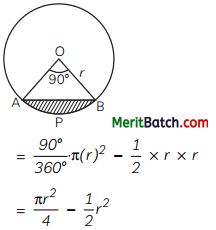Question 7.
What is the volume of tire material in a spherical shell with inner radius ‘r’ and outer radius ‘R’ ? 
(a) $$\frac{4}{3}$$π(R3 – r3)
(b) π(R3 – r3)
(c) $$\frac{1}{2}$$(R3 – r3)
(d) None of these
(a) $$\frac{4}{3}$$π(R3 – r3)

Explanation:
Volume of material in a spherical shell
= $$\frac{4}{3}$$π(R3 – r3)Question 8.
If tan θ = 1, then the value of sec θ + cosec θ is: 
(a) 3√2
(b) 4√2
(c) 2√2
(d) √2
(c) 2√2

Explanation:
Given, tan θ = 1, we have θ = 45°
So, sec θ + cosec θ = √2 + √2 = 2√2.

Question 9.
If 3 tan2x = 1 (0° < x < 90°), then what is the value of x. 
(a) 60°
(b) 90°
(c) 30°
(d) 45°
(c) 30°

Explanation:
Given: 3 tan2x = 1
⇒ tan2x = $$\frac{1}{3}$$
⇒ tan x = $$\frac{1}{\sqrt{3}}$$
⇒ x = 30°.

Question 10.
What is the positive real root of 64x2 – 1 = 0? 
(a) $$\frac{1}{8},-\frac{1}{8}$$
(b) $$\frac{1}{4},-\frac{1}{4}$$
(c) $$\frac{1}{2}, \frac{1}{3}$$
(d) $$\frac{1}{6}, \frac{-1}{7}$$
(a) $$\frac{1}{8},-\frac{1}{8}$$

Explanation:
Given,
⇒ 64x2 – 1 = 0
⇒ (8x + 1) (8x – 1) = 0
⇒ 8x = – 1, 8x = 1
⇒ x = $$\frac{1}{8},-\frac{1}{8}$$
Thus, positive root is $$\frac{1}{8}$$

Question 11.
The radii of 2 cylinders are in the ratio 2 : 3 and their heights are in the ratio 5 : 3. Then, the ratio of their volumes is: 
(a) 19 : 20
(b) 20 : 27
(c) 18:25
(d) 17:23
(b) 20 : 27

Explanation:
Let r1 and r2 be the two radii and hi and h2 be the corresponding two heights of the two cylinders. Then
$$\frac{r_1}{r_2}=\frac{2}{3}$$ (Given)
and $$\frac{h_1}{h_2}=\frac{5}{3}$$ (Given)
So, $$\frac{V_1}{V_2}=\frac{\pi r_1^2 h_1}{\pi r_2^2 h_2}=\frac{4}{9} \times \frac{5}{3}=\frac{20}{27}$$
Thus, V1 : V2 = 20 : 27.

Question 12.
If a and p be the zeros of the quadratic polynomial 2x2 + 5x + 1, then the value of α + β + αβ is: 
(a) -2
(b) 2
(c) -3
(d) 4
(a) -2

Explanation:
Here, α + β = $$-\frac{5}{2}$$; αβ = $$\frac{1}{2}$$
So, α + β + αβ = $$-\frac{5}{2}+\frac{1}{2}$$ = – 2.

Question 13.
An integer is chosen at random between 1 and 100. The probability that chosen number is divisible by 10 is: 
(a) $$\frac{2}{9}$$
(b) $$\frac{1}{100}$$
(c) $$\frac{1}{5}$$
(d) $$\frac{1}{50}$$
(b) $$\frac{1}{100}$$

Explanation:
Required probability = $$\frac{\text { Favourable cases }}{\text { Total no. of cases }}$$
= $$\frac{10}{100}=\frac{1}{10}$$

Question 14
In the figure, if $$\frac{O A}{O D}=\frac{O C}{O B}$$, thenwhich pair of angles are equal? 
(a) ∠A = ∠C
∠B = ∠D
(b) ∠A = ∠B
∠C = ∠D
(c) ∠C = ∠B
∠A = ∠D
(d) None of these
(a) ∠A = ∠C
∠B = ∠D

Explanation:
In given figure, $$\frac{O A}{O D}=\frac{O C}{O B}$$ or $$\frac{O A}{O C}=\frac{O D}{O B}$$
Then, in ΔAOD and ΔCOB
$$\frac{O A}{O C}=\frac{O D}{O B}$$
and ∠AOD = ∠COB
(vertically opposite angles)
ΔAOD ~ ΔCOB
∠A = ∠C and ∠B = ∠D.

Question 15.
Root of the equation x2 – 0.04 = 0 is: 
(a) 2
(b) 0.02
(c) 4
(d) ±0.2 1
(d) ±0.2 1

Explanation:
A root of x2 – 0.04 = 0
x2 = 0.04
x = $$\sqrt{0.04}$$
x = ± 0.2Question 16.
If 6 times the 6th term of an A.P., is equal to 9 times the 9th term, find its 15th term is: 
(a) 65
(b) 0
(c) 46
(d) 1
(b) 0

Explanation:
Let, the first term of A.P. be ‘a’ and its common difference be ‘d’
Given, 6a6 = 9a9
⇒ 6(a + 5d) = 9(a + 8d)
⇒ 6a + 30d = 9a + 72d
⇒ -3a = 42d
⇒ a = -14d …(i)
Then, 15th term i.e. a15 = a + 14d
= -14d + 14d [from (i)]
= 0
Hence, 15th term of A.P. is 0.

Question 17.
The solution of the following pair of equation is: 
x – 3y = 2, 3x – y = 14
(a) x = 5, y = 1
(b) x = 2, y = 3
(c) x = 1, y = 2
(d) x = 1, y = 4
(a) x = 5, y = 1

Explanation:
Given, equations are:
x – 3y = 2 …(i)
and 3x – y = 14 …(ii)
Multiplying equation (i) by 3 and then subtracting equation (ii) from it, we get⇒ y = 1
x = 2 + 3y
= 2 + 3 × 1 = 5
Hence, x = 5 and y = 1

Question 18.
The chord of a circle of radius 8 cm subtends a right angle at its centre. The length of the chord is: 
(a) 8 cm
(b) 8√2 cm
(c) 7 cm
(d) 7√2 cm
(b) 8√2 cm

Explanation:
Since, ∠AOB = 90°∴ By Pythagoras theorem
AB2 = OA2 + OB2
= 82 + 82
= 64 + 64 = 128
AB = $$\sqrt{128}$$
= 8√2 cm

Direction for questions 19 and 20: In question number 19 and 20, a statement of Assertion (A) is followed by a statement of Reason (R).
Choose the correct option:
(a) Both assertion (A) and reason (R) are true and reason (R) is the correct explanation of assertion (A)
(b) Both assertion (A) and reason (R) are true but reason (R) is not the correct explanation of assertion (A)
(c) Assertion (A) is true but reason (R) is false.
(d) Assertion (A) is false but reason (R) is true.

Question 19.
Assertion (A): The HCF of two numbers is 9 and their LCM is 2016. If the one number is 54, then the other number is 336.
Reason (R) : Relation between numbers and their HCF and LCM is product of two numbers a, b = HCF (a, b) × LCM (a, b). 
(a) Both assertion (A) and reason (R) are true and reason (R) is the correct explanation of assertion (A)

Explanation:
Let the other number be x.
9 × 2016 = 54 × x
⇒ x = 336Question 20.
Assertion (A): A 28 cm-diameter semicircle of paper is bent into a conical cup shape that is open at the top. The cup’s radius is 7cm.
Reason (R) : Circumference of base of conical cup = 1/2x circumference of arc of semi-circular sheet. 
(a) Both assertion (A) and reason (R) are true and reason (R) is the correct explanation of assertion (A)

Explanation:
Let the radius of the conical cup be R.
Then, circumference of base of conical cup
= $$\frac{1}{2}$$ × Circumference of arc of semi-circular sheet
⇒ 2πR = $$\frac{1}{2}$$ × 2πr
⇒ 2R = $$\frac{28}{2}$$
⇒ R = $$\frac{28}{4}$$ = 7 cm

SECTION – B (10 marks)
(Section – B consists of 5 questions of 2 marks each.)

Question 21.
Find the HCF of 72 and 120, using prime factorisation method. 
OR
Write a pair of equations in variables x and y which is consistent with:
(A) unique solution
(B) infinitely many solutions.
Here,
72 = 2 × 2 × 2 × 3 × 3, or 23 × 32
120 = 2 × 2 × 2 × 3 × 5, or 23 × 31 × 51
So, HCF(72, 120) = 23 × 31 , i.e. 24
OR
(A) x + 3y – 5 = 0; 3x + 6y – 2 = 0
Here, $$\frac{1}{3} \neq \frac{3}{6} \neq \frac{-5}{-2}$$

(B) x + 3y – 5 = 0; 2x + 6y – 10 = 0
Here, $$\frac{1}{3} \neq \frac{3}{6} \neq \frac{-5}{-2}$$

Question 22.
Determine the value(s) of k for which the quadratic equation 4x2 – 3kx + 9 = 0 has no real roots. 
For the given equation, we have Discriminant,
D = (-3k)2 – 4(4)(9)
= 9k2 – 144
Equation will have no real roots, when D < 0
i.e. 9k2 – 144 < 0
or k2 < 16
⇒ – 4 < k < 4.

Question 23.
In a school, students thought of planting 250 trees in and around the school to reduce air pollution. It was decided that the number of trees, that each section of each class will plant, will be the same as the class in which they are studying, e.g., a section of Class I will plant 1 tree, a section of Class II will plant 2 trees and so on till class XII (there are three sections of each class). The remaining number of trees will be planted by teachers of the school.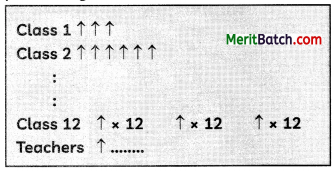(School Tree Plantations)
(A) Determine the number of trees that will be planted by Class VII and by Class X.
(B) Determine the number of trees that will be planted by students of the school.
Class 1 ↑
Class 2 ↑ ↑
.
.
Class 12↑ × 12 ↑ × 12 ↑ × 12
Obtained series, 3, 6, 9, ……….. 36
The above series is an A.P. with a = 3 and d = 3.
(A) The number of trees planted by class VII
a + 6d = 3 + 6(3) = 21
The number of trees planted by class X
a + 9d = 3 + 9(3) = 30

(B) The number of trees planted by students
= $$\frac{n}{2}$$ [2a + (n – 1) d]
= $$\frac{12}{2}$$ [2 x 3 + (12 – 1) x 3]
= 6 [6 + 33]
= 6 (39)
= 234

Question 24.
With his friends, Siddharth visited a park where he sat behind a tree next to a lovely lamp post AB. His friends estimated that the approximate distance between the lamp post’s foot and the tree’s foot was 7 metres. If AC + AB = 49 m , then find the value of sec C.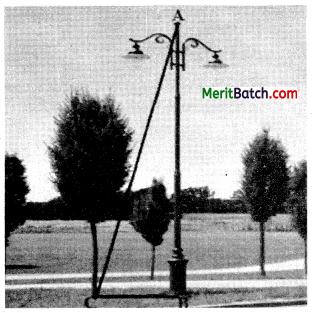OR
The vertices of an equilateral triangle ABC are (0, 0) (0, y) and (3, √3), then find the value of y. 
It is given that, BC = 7 m and AC + AB = 49 m,
which can be written as AB = (49 – AC)
As ΔABC is a right triangle, right angled at B, we will apply Pythagoras theorem to find the measures of unknown sides.
According to Pythagoras theorem,
AC2 = AB2 + BC2 = (49 – AC)2 + 49
= 2401 – 98 AC + AC2 + 49

Solving, we get, 98AC = 2450
AC = 25 m
Therefore, AB = 49 – 25 = 24 m.
We know that sec C = $$\frac{\text { hypotenuse }}{\text { base }}=\frac{25}{7}$$
OR
Since, A ABC is an equilateral triangle,
∴ AB = BC = AC
or AB2 = BC2 = AC2
or AB2 = AC2∴ By distance formula
⇒ (0 – 0)2 + (0 – y)2 = (0 – 3)2 + (0 – 43 )2
⇒ y2 = 9 + 3
⇒ y2 = 12
⇒ y = $$\sqrt{12}$$ = ± 2√3 .

Question 25.
A dice is thrown twice. What is the probability of getting a doublet? 
When a dice is thrown twice, the total number of probable cases are 36 and favourable cases of getting a doublet are (1, 1) (2, 2) (3, 3) (4, 4) (5, 5) (6, 6) i.e., number of favourable cases is 6.
∴ Required probability = $$\frac{6}{36}$$
= $$\frac{1}{6}$$

SECTION – C (18 marks)
(Section – C consists of 6 questions of 3 marks each)

Question 26.
Prove that
$$\frac{\cos ^2 \theta}{1-\tan \theta}+\frac{\sin ^2 \theta}{1-\cot \theta}$$ = 1 + sin θ + cos θ
OR
If cos θ + sin θ = √2 cos θ, show that cos θ – sin θ = √2 sin θ.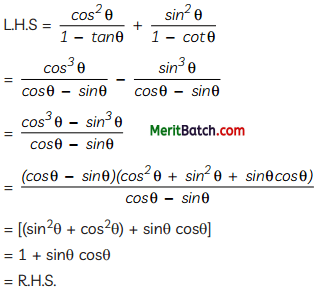OR

Given, cos θ + sin θ = √2 cos θ
Squaring both sides, we get
(cos θ + sin θ)2 = (√2 cos θ)2
⇒ cos2θ + sin2θ + 2sin θ cos θ
⇒ 2sin θ cos θ = cos2θ – sin2θ
⇒ 2sin θ cos θ = (cos θ – sin θ)(cos θ + sin θ)
⇒ 2sin θ cos θ = (cos θ – sin θ) (√2 cos θ)
⇒ √2 sin θ = cos θ – sin θ
or cos θ – sin θ = √2 sin θ
Hence Proved.Question 27.
In the figure, chord AB subtends an angle of 60° at the centre O of the circle of radius 3.5 cm. Find the:
(A) length of the arc APB
(B) the area of the sector AOB
(C) area of the minor segment (Shaded region) (Use √3 = 1.73)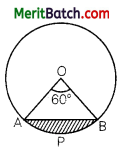OR
In the figure, ABC is an isosceles triangle with BC = 8 cm and AB = AC = 5 cm.Find:
(A) sin B
(B) tan C
(C) sin2B + cos2C
(A) Length of arc APB = $$\frac{60^{\circ}}{360^{\circ}}$$ × 2π × (3.5) cm = $$\frac{11}{3}$$ cm

(B) Here, area of the sector AOB
= $$\frac{60^{\circ}}{360^{\circ}}$$ × π × (3.5)2
= $$\frac{77}{12}$$ sq.cm

(C) Area of the minor segment
= Area of sector AOB – Area of ΔAOB
= [$$\frac{77}{12}$$sq.cm – $$\frac{\sqrt{3}}{4}$$(3.5)2sq. cm]
= $$\left(\frac{77}{12}-\frac{53}{10}\right)$$ sq.cm
= $$\frac{67}{60}$$ sq.cm

OR

ΔABC is isoscel.es. So, altitude AD bisects side BC.
Thus, BD = DC = 4cm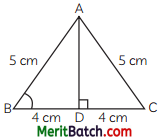AD = $$\sqrt{A B^2-B D^2}=\sqrt{5^2-4^2}$$
= $$\sqrt{25-16}=\sqrt{9}$$ = 3 cm.
So,
(A) sin B = $$\frac{A D}{B A}=\frac{3}{5}$$
(B) tan C = $$\frac{A D}{D C}=\frac{3}{4}$$
(C) sin2B + cos2C = $$\left(\frac{3}{5}\right)^2+\left(\frac{4}{5}\right)^2$$
= $$\frac{9}{25}+\frac{16}{25}=\frac{25}{25}$$ = 1

Question 28.
If sin A + cos A = p and sec A + cosec A = q, then prove that q(p2 -1) = 2p. 
Here, p = sin A + cos A; q = sec A + cosec A So, q (p2 – 1)
= (sec A + cosec A) [(sin A + cos A)2 – 1]
= (sec A + cosec A) [(sin2 A + cos2 A + 2 sin A cos A) – 1]
= (sec A + cosec A) [ 1 + 2 sin A cos A – 1] {since sin2 A + cos2 A = 1}
= (sec A + cosec A) (2 sin A cos A)
= 2 sin A cos A sec A + 2 sin A cos A cosec A
= 2 sin A + 2 cos A
= 2 (sin A + cos A)
= 2p.
Hence Proved.Question 29.
Let A(4, 2), B(6, 5) and C(1, 4) be the vertices of ΔABC. The median AD from A meets BC in D. Find the coordinates of the point P on AD such that AP: PD = 2 :1. 
Here, D is D$$\left(\frac{6+1}{2}, \frac{5+4}{2}\right)$$ i.e, D$$\left(\frac{7}{2}, \frac{9}{2}\right)$$

The Point P divides AD in the ratio 2 : 1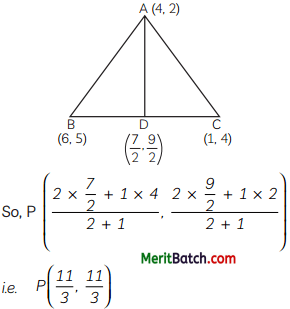Question 30.
The fifth, sixth, and seventh-grade kids at the school will participate in a webinar about cgbercrime awareness. He requested that the teachers incharge of these classes join all the students in one room. 84 kids are enrolled in fifth grade, 63 in sixth grade, and 42 in seventh grade. What is minimum number of rows in which the students can be seated so that students of same class sit in a same row?
Here, numbers are 84, 63 and 42
So, we need to find their HCF.
∴ 84 = 22 × 3 × 7
63 = 32 × 7
42 = 2 × 3 × 7
∴ HCF = 3 × 7
= 21
∴ Number of rows for class fifth = $$\frac{84}{21}$$ = 4
∴ Number of rows for class sixth = $$\frac{63}{21}$$ = 3
∴ Number of rows for class seventh = $$\frac{42}{21}$$ = 2
∴ Total number of rows = 4 + 3 + 2 = 9

Question 31.
One card is drawn from a well shuffled pack of 52 cards, each of which is equally likely to be Draw. Find the probability that the card drawn is: 
(A) either black or a queen
(B) black and a queen
(C) neither a jack nor a king.
(A) P(either black or a queen card) $$\frac{28}{52}$$ i.e $$\frac{7}{13}$$
[There are 26 black cards and two queens of red colour]

(B) P(black and a queen) = $$\frac{2}{52}$$ i.e. $$\frac{1}{26}$$
[There are only 2 black queens]

(C) P(neither a jack nor a king) = $$\frac{44}{52}$$ i.e. $$\frac{11}{13}$$
[There are 4 jacks and 4 kings in the pack of 52 cards. So, neither jack nor king cards = 52 – 4 – 4 = 44]

SECTION – D (20 marks)
(Section – D consists of 4 questions of 5 marks each.)

Question 32.
In the figure, ABC and DBC are two triangles on the same base BC. If AD intersects BC at O,Show that: $$\frac{{ar}(\triangle A B C)}{{ar}(\triangle D B C)}=\frac{A O}{D O}$$
OR
Prove that the line segments joining the mid-points of the sides of a triangle form four triangles, each of which is similar to the original triangle. 
Draw AM ± BC and DN ± BC
In Δs AOM and DON, we have:
∠M = ∠N [Each 90°]
∠AOM = ∠DON
[Vertically opposite angles]So, by AA similarity criterion,
ΔAOM ~ ΔDON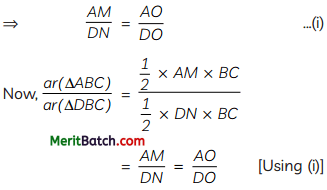OR
Consider a triangle ABC in which D, E, F are respectively the mid-points of sides BC, CA, AB.
Now, since E and F are mid-points of AB and AC respectively.
So, by mid-point theorem, we have
FE ∥ BC
∠AFE = ∠B
[Corresponding angles]Thus, in ΔAFE and ΔABC. we have:
∠AFE = ∠B [Proved abovel
and ∠A = ∠A [Common angLe]
So, ΔAFE ~ ΔABC
Similarly,
ΔFBD ~ ΔABC
and ΔEDC ~ ΔABC
Now, we shall show that ΔDEF ~ ΔABC
Clearly, ED ∥ AF and DF ∥ EA
AFDE is a parallelogram.
⇒ ∠EDF = ∠A
Similarly, BDEF is a parallelogram.
∴ ∠DEF = ∠B
Thus, in As DEF and ABC, we have:
∠EDF = ∠A, ∠DEF = ∠B
So, by AA similarity criterion, ΔDEF ~ ΔABC
Thus, each one of the triangles AFE, FBD, EDC and DEF is similar to ΔABC.
Hence Proved.Question 33.
From the top of a house, which is at a height of 25 m from the level ground, a man observes the top of a building at an angle of elevation of 30° and angle of depression of the base of the ^ building to be 60°. Calculate the height of building. 
Here, EC be a building and AB be a house.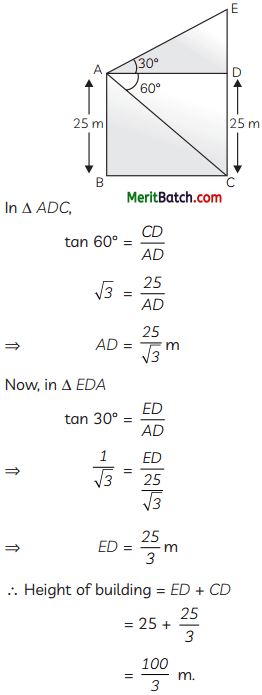Question 34.
PQ and QR are tangents to the circle in the given figure, with centre O, at P and R respectively. Find the value of x.∠OPQ = ∠ORQ = 90°
(As tangent is 1 to the radius)
∠ROP = 360° – (50° + 90° + 90°) = 360° – 230°
= 130°
reflex ∠ROP = 360° – 130°
= 230°

In DROS, OR = OS (radii of the circle)
∴ ∠RSO = ∠ORS = 20°
Now, ∠ROS = 180° – (20° + 20°)
= 140°
[By angle sum property]
∠POS = reflex ∠ROP – ∠ROS
= 230 – 140 = 90°
In DPOS (PO = OS, radii of circle)
∠OPS = ∠OSP = 45°
∠OPT = 90°
(as tangent is ⊥ to the radius)
∴ x = 90° – 45 = 45°

Question 35.
Three kg of wheat and one kg of pulse cost ₹ 96 last year. Pulse prices have grown by 15% this year, while wheat prices have gone up by 10%. The same quantity of wheat and pulse therefore cost ₹ 108.60. Find the wheat and pulse per kg prices from the last year.
OR
After calculating the zeroes of the quadratic polynomial p(x) = abx2 + (b2 – ac)x – be, verify the relationship between them and its coefficients. 
Let, the rate of pulse last year be ₹ x/kg and rate of wheat last year be ₹ y/kg
ATQ,
x + 3y = 96 ……..(i)
⇒ $$\frac{115}{2}$$x + $$\frac{3 \times 110}{100}$$y = 108.60
⇒ $$\frac{23 x}{20}+\frac{3 \times 22 y}{20}$$ = 108.60
⇒ 23x + 66y = 108.60 × 20
⇒ 23x + 66y = 2172 …(ii)

Multiply eqn. (i) by 22 subtract (i) from (ii)Put the value of x in eqn. (i)
60 + 3y = 96
3y = 36
y = 12
Hence, the rate of mixed pulse last year was ₹ 60/kg and that of wheat was ₹ 12/kg.
OR
Given, quadratic polynomial is: p(x) = abx2 + (b2 – ac)x – bc
Let a and b be the zeroes of the quadratic polynomial p(x).
∴ Sum of zeroes, α + β = $$\frac{-\left(b^2-a c\right)}{a b}$$
= $$\frac{a c-b^2}{a b}$$
Product of zeroes, αβ = $$\frac{-b c}{a b}=\frac{-c}{a}$$
By equation;
p(x) = abx2 + b2x – acx – bc
= bx (ax + b) – c(ax + b)
= (bx – c) (ax + b)

For finding zeroes, put p(x) = 0
(bx – c) (ax + b) = 0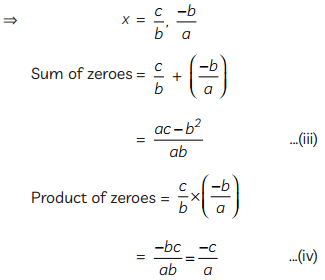From (i), (ii), (iii) and (iv), the relation is verified.

SECTION – E (12 marks)
(Case Study-Based Questions)
(Section – E consists of 3 questions. All are compulsory.)

Question 36.
One very useful application of Integration is finding the area and volume of “curved” figures, that we couldn’t typically get without using Calculus. Although there is no need to know anything about that topic, Mrs Meena wanted to use the concept to help students figure out how area of an enclosed surface, that could be easily calculated using parabolic curve.
The diagram shows a sketch of the curve y = 36 – x2. The curve crosses the axes at H, K and L. O is the origin.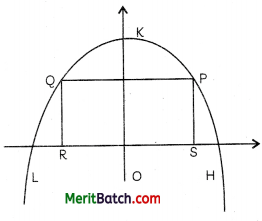The point P be P(x, y) and A be the area of the rectangle PQRS.
On the basis of the above information, answer the following questions:
(A) Find the area of the triangle OHK.
OR
The area A of the rectangle PQRS and find the value of A at x = 3. 
(B) Find the non – zero value of x for which A = 0. 
(C) Find the length of line segment HK. 
(A) For the curve y = x2 – 36.
Since the point H lies on the curve as well as on the x-axis,
So, for the point H,
y = 0
⇒ 36 – x2 = 0
⇒ x2 = 36
⇒ x = ± 6
Since point H lies on positive side of x-axis
H = (6, 0)
Similarly for the point K,
x = 0
y = 36 – (0)2
y = 36
K = (0, 36)
ΔOHK = $$\frac{1}{2}$$ × OH × QK
= $$\frac{1}{2}$$ × 6 × 36
So, area of ΔOHK = 108 sq. units

OR

A = PQ × PS
= 2y × x
= 2(36 – x2)x
= 72x – 2x3
At x = 3,
A = 72 × 3 – 2(3)3
= 216 – 54 = 162

(B) When A = 0,
72x – 2x3 =0
2x3 = 72x
= 36
x=±6

(C) Using distance formula,
HK = $$\sqrt{(O H)^2+(O K)^2}$$
= $$\sqrt{(6-0)^2+(36-0)^2}$$
= $$\sqrt{36+1296}$$
= $$\sqrt{1332}$$
= 36.49 36.5 unitsQuestion 37.
Google maps cartography team is working on improving the scalability quality of maps when you use the app on your phones to zoom in using 4 fingers. They are using a proprietary tool called ‘MapMaker’ to figure out scalability factors. A mathematical model is created for a type of object (below cross-section) to test its scalability on maps app.In the diagram, AC = 8 cm, CE = 4 cm and the area of the triangle BEC is 4.2 sq cm.
Another enlargement with centre E, mapsOn the basis of the above information, answer of the following questions:
(A) An enlargement , with centre A, maps ΔABC onto ΔADE, then find the scale factor of the enlargement. 
(B) Find the length of AF. 
(C) Find the area of ΔEFA.
OR
Find the area of ΔBAF. 
(A) Scale factor = $$\frac{A C}{A E}$$
= $$\frac{A C}{A C+C E}=\frac{8}{8+4}$$
= $$\frac{8}{12}=\frac{2}{3}$$

(B) Since, ΔEBC ~ ΔEAF
$$\frac{E C}{E A}=\frac{B C}{A F}$$
$$\frac{4}{12}=\frac{3.6}{A F}$$
⇒ AF = 3.6 × 3
= 10.8 cm

(C) Since, with centre E enlargement as done to DEBC and DEFA.
∴ ΔEBC ~ ΔEFF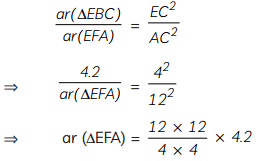= 3 × 3 × 4.2
= 37.8 sq. cm

OR

area of ΔBAF = $$\frac{1}{2}$$ × AF × AB
= $$\frac{1}{2}$$ × 10.8 × 7.15
= 38.61 sq. cm

Question 38.
The best way to determine a loaf’s freshness is to see how it responds to a sharp serrated knife. Freshly baked loaves will be difficult to slice because they’re full of moisture. When bread dries out, it becomes easier to slice since it keeps its shape better—up to a point. When bread becomes truly stale, it will be so hard that it’s difficult to slice. A bread manufacturer wants to know the lifetime of the product. For this, he tested the life time of 400 packets of bread. The following tables gives the distribution of the life time of 400 packets.

 Lifetime (in hours) Number of packets (Cumulative frequency) 150-200 14 200-250 70 250-300 130 300-350 216 350-400 290 400-450 352 450-500 400On the basis of the above information, answer the following questions:
(A) If m be the class mark and b be the upper limit of a class in a continuous frequency distribution, then find the lower limit of the class. 
(B) Find the average life time of a packet.
OR
Find the median life time of a packet. 
(C) If empirical formula is used, then find the modal life time of a packet. 
(A) Class mark = $$\frac{\text { Lower limit }+\text { Upper limit }}{2}$$
⇒ m = $$\frac{\text { Lower limit }+b}{2}$$
⇒ Lower limit = 2m – b

(B)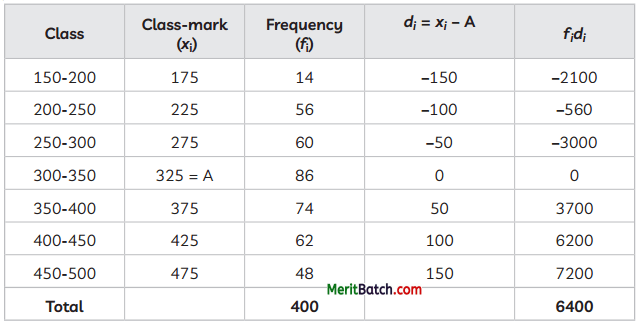∴ Average Lifetime of a packet
= A + $$\frac{\Sigma f_i d_i}{\Sigma f_i}$$ = 325 + $$\frac{6400}{400}$$ = 341 hrs

OR
Also, cumulative frequency for the given distribution are 14, 70, 130, 216, 290, 352, 400
∴ c.f just greater than 200 is 216, which is corresponding to the intervaL 300-350.
l = 300, f = 86, cf = 130, h = 50

∴Median =
l + $$\left(\frac{\frac{N}{2}-c . f}{f}\right)$$ × h = 300 + $$\left(\frac{\frac{N}{2}-c . f}{f}\right)$$ × 50
= 300 + 40.697 = 340.697 ≈ 340 hrs (approx.)

(C) We know that,
Mode = 3 Median – 2 Mean
= 3(340.697) – 2(341)
= 1022.091 – 682
= 340.091 ≈ 340 hrs